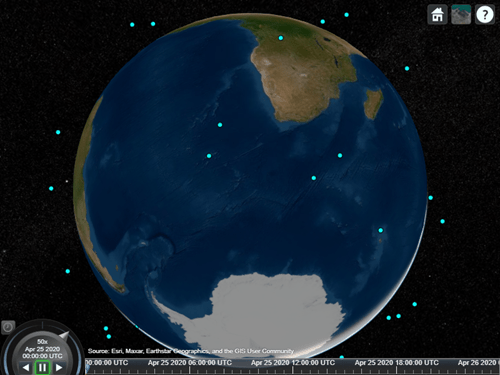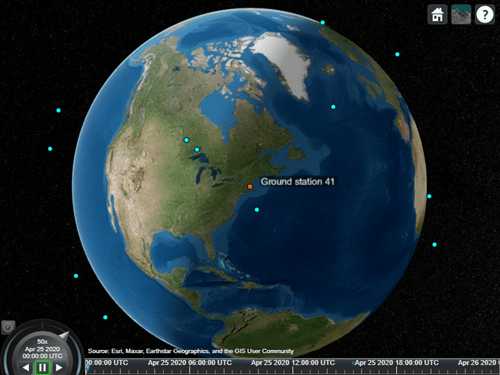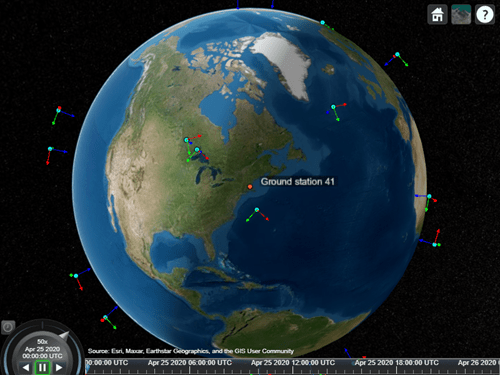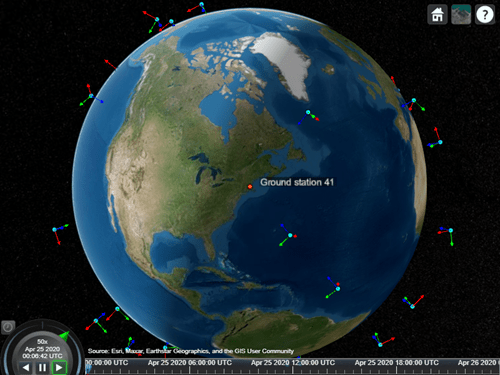# coordinateAxes

Visualize coordinate axes triad of satellite scenario assets

Since R2023a

## Syntax

``coordinateAxes(asset)``
``coordinateAxes(asset,Name=Value)``
``ax = coordinateAxes(___)``

## Description

example

````coordinateAxes(asset)` adds a coordinate axes triad graphic visualization (`CoordinateAxes` object) for an asset. In the visualization, the x-axis (roll) is red in color, the y-axis (pitch) is green, and z-axis (yaw) is blue.```
````coordinateAxes(asset,Name=Value)` specifies additional arguments using one or more name-value.```
````ax = coordinateAxes(___)` returns the `CoordinateAxes` object.```

## Examples

collapse all

Create a `satelliteScenario` object.

```startTime = datetime(2020,4,25); % April 25, 2020, 12:00 AM UTC stopTime = datetime(2020,4,26); % April 26, 2020, 12:00 AM UTC sampleTime = 60; % seconds sc = satelliteScenario(startTime,stopTime,sampleTime);```

Launch a satellite scenario viewer with `ShowDetails` set to false.

`v = satelliteScenarioViewer(sc,ShowDetails=false);`Add a low-earth orbit satellite constellation to the scenario from a TLE file.

```tleFile = "leoSatelliteConstellation.tle"; sat = satellite(sc,tleFile);```Add a ground station to the scenario.

```gs = groundStation(sc); gs.ShowLabel = true;```Visualize the coordinate axes triads of the satellites.

`ax = coordinateAxes(sat)`
```ax=1×40 object 1×40 CoordinateAxes array with properties: Scale VisibilityMode ```

Point the satellites towards the ground station. The coordinate axes triad object updates to represent the new direction of the satellites.

`pointAt(sat,gs);`Play the scenario.

`play(sc); `## Input Arguments

collapse all

Satellites, ground stations, gimbals, conical sensors, transmitters, or receivers, specified as a vector.

### Name-Value Arguments

Specify optional pairs of arguments as `Name1=Value1,...,NameN=ValueN`, where `Name` is the argument name and `Value` is the corresponding value. Name-value arguments must appear after other arguments, but the order of the pairs does not matter.

Example: `Scale =2` scales the size of the coordinate axes triad visualization by 2.

Satellite scenario viewer, specified as a vector. You view the coordinate axes triad in the viewer specified in this argument.

Scale of the coordinate axes triad graphic, specified as a positive scalar.

Data Types: `double`

## Output Arguments

collapse all

Coordinate axes triad, returned as an object.

## Version History

Introduced in R2023a